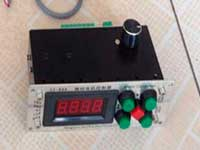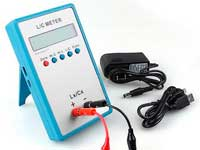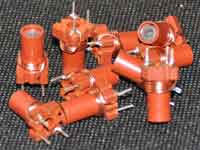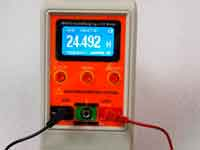Multilayer inductor on a rectangular form - online calculator - 5.0 out of 5 based on 4 votes
Rating 5.00 (4 Votes)

Multilayer air core inductor on a rectangular former:The calculator allows calculating the number of windings of the multilayer air core coil on a rectangular former at the design stage. You can select measurement units - mm or inches, and also AWG or SWG wire number - the wire diameter will be determined automatically. This calculator based on Rosa's straight wire self-inductance formula (formula 9 on p.305) and formula for mutual-inductance between two unequal straight wires from American National Bureau of Standards circular C74 (formula 182 p.273 with error correction). The coil is represented as many parts of straight wires. Calculating the self-inductance of each part, and mutual inductance of each possible pair of straight wire parts and summing it all, we get a self-inductance of the multilayer coil.

This is the "virtual winding numerical method" that is the same as in the calculation of multilayer coil with round former. The accuracy of the calculation is to 5%. At a very high value of inductance, it's possible the small hang. See more about source formulas. Many thanks to Robert Weaver for his great help.

Calculate number of turnsENTER THE INPUT DATA:
 Select units: mm/cmmil/inch AWG → 000000000012345678910111213141516171819202122232425262728293031323334353637383940 SWG → 7/06/05/04/03/02/001234567891011121314151617181920212223242526272829303132333435363738394041424344454647484950

 L = mHμHnH – Required inductance a – Width of former cross-section b – Height of former cross-section l – Length of winding (former) d – Diameter of wire without insulation k – Diameter of wire with insulation Automatic

RESULT:
 N = – Number of turns n = – Number of Layers c – Winding thickness Lw – Required length of wire Ω =  Ohm – DC Resistance of coil

Another useful calculator:

Сomments from anonymous guests are enabled with moderation.

Coil Winding MachineUS \$187.53
The system consists of individual components including a controller, a stepping motor, a foot switch, power adapter and output shaft. This design makes it very easy for you to integrate the system into your own project.

LC Meter LC200AUS \$37.99
Measuring Capacitance Range:
0.01 pF - 100mF
Measuring Inductance Range:
0.001 uH - 100H
Measurement accuracy:1%.

RF InductorsUS \$6.43
Variable Ferrite Core Inductor coil set 3.5Turns 25-100MHZ

Auto Ranging LCR MeterUS \$63.07
Meter can measure 0.00pF to 100.00mF capacitance, 0.000uH to 100.00H inductance and 0.00? to 10.000M? resistance. Very suitable for small value capacitor and inductor measurement.